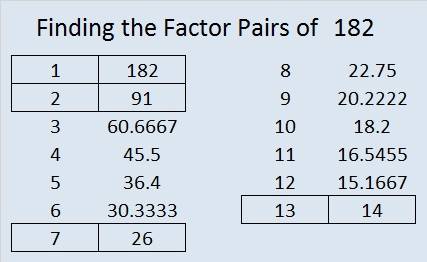# 182 and Level 3

 182 is a composite number. Factor pairs: 182 = 1 x 182, 2 x 91, 7 x 26, or 13 x 14 Factors of 182: 1, 2, 7, 13, 14, 26, 91, 182 Prime factorization: 182 = 2 x 7 x 13Notice this for 182: 18 – (2 x 2) = 18 – 4 = 14, a multiple of 7, so 182 can be evenly divided by 7.

A Logical Approach to FIND THE FACTORS: Find the column or row with two clues and find their common factor. Write the corresponding factors in the factor column (1st column) and factor row (top row).  Because this is a level three puzzle, you have now written a factor at the top of the factor column. Continue to work from the top of the factor column to the bottom, finding factors and filling in the factor column and the factor row one cell at a time as you go.